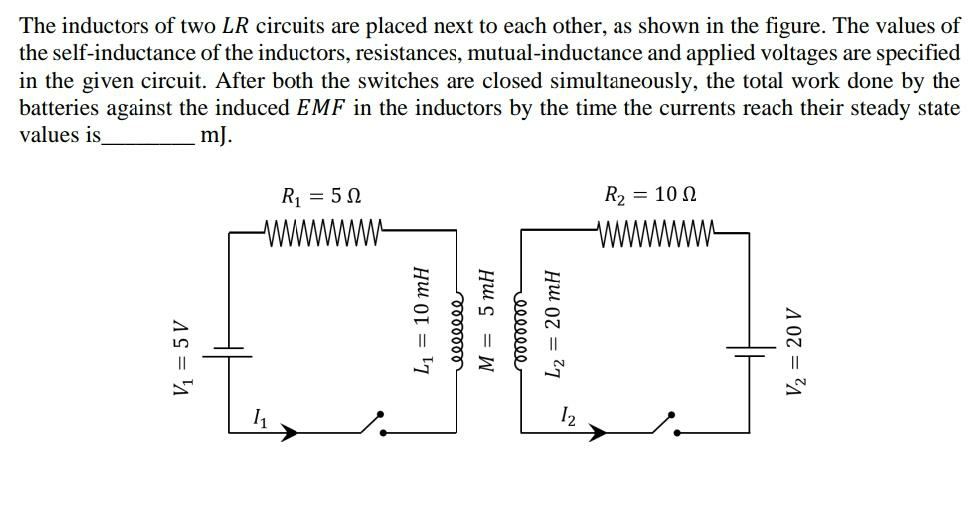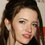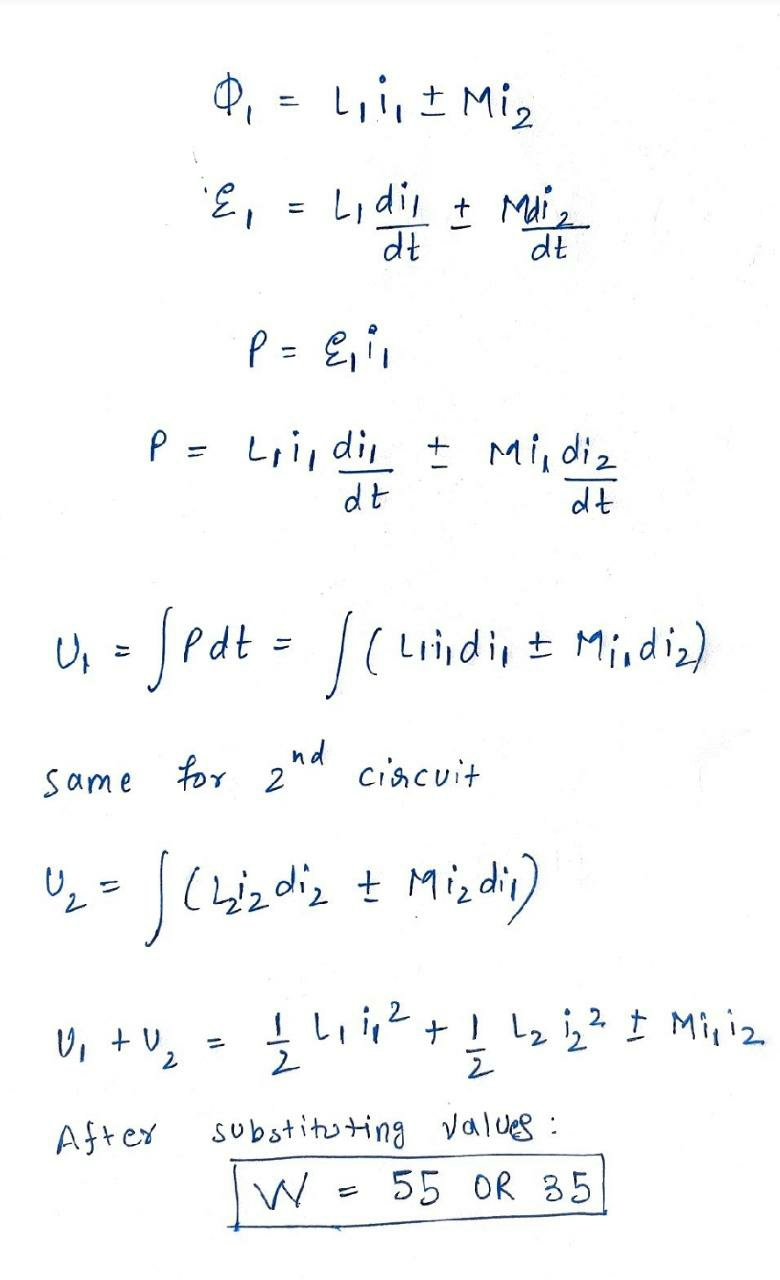# Famous Exam ProblemNote by Talulah Riley
10 months ago

This discussion board is a place to discuss our Daily Challenges and the math and science related to those challenges. Explanations are more than just a solution — they should explain the steps and thinking strategies that you used to obtain the solution. Comments should further the discussion of math and science.

When posting on Brilliant:

• Use the emojis to react to an explanation, whether you're congratulating a job well done , or just really confused .
• Ask specific questions about the challenge or the steps in somebody's explanation. Well-posed questions can add a lot to the discussion, but posting "I don't understand!" doesn't help anyone.
• Try to contribute something new to the discussion, whether it is an extension, generalization or other idea related to the challenge.

MarkdownAppears as
*italics* or _italics_ italics
**bold** or __bold__ bold
- bulleted- list
• bulleted
• list
1. numbered2. list
1. numbered
2. list
Note: you must add a full line of space before and after lists for them to show up correctly
paragraph 1paragraph 2

paragraph 1

paragraph 2

[example link](https://brilliant.org)example link
> This is a quote
This is a quote
    # I indented these lines
# 4 spaces, and now they show
# up as a code block.

print "hello world"
# I indented these lines
# 4 spaces, and now they show
# up as a code block.

print "hello world"
MathAppears as
Remember to wrap math in $$ ... $$ or $ ... $ to ensure proper formatting.
2 \times 3 $2 \times 3$
2^{34} $2^{34}$
a_{i-1} $a_{i-1}$
\frac{2}{3} $\frac{2}{3}$
\sqrt{2} $\sqrt{2}$
\sum_{i=1}^3 $\sum_{i=1}^3$
\sin \theta $\sin \theta$
\boxed{123} $\boxed{123}$

Sort by:

@Steven Chase Have a look. And also if possible, tell me the time you take to solve this. Thanks in advance.

- 10 months ago

One more thing , please solve anaylitcally.

- 10 months ago

- 10 months ago

My attempt. I have not solved it completely.

Circuit equations are:

$-V_1 + L_1 \dot{I}_1 + M\dot{I}_2 + I_1R_1=0$ $-V_2 + L_2 \dot{I}_2 + M\dot{I}_1 + I_2R_2=0$

Now, $I_1(0) = I_2(0)=0$. You need to solve the above set of differential equations and use that solution to compute the work done as follows:

$W = \int_{0}^{t_f} (V_1I_1+V_2I_2) \ dt$

- 10 months ago

@Karan Chatrath we can solve without solving differential equation also .I have solved it. It is a jee advance 2020 problem. A very fun problem

- 10 months ago

@Karan Chatrath Here is the solution. In case you interested- 10 months ago

I do not think this is correct. This is because you have assumed that all work done by the battery is energy stored in the inductors. There is heat dissipated in the resistors also.

- 10 months ago

@Karan Chatrath I am using the formula for battey only $P=Vi$ and intergrating $p$ w.r.t $dt$. , Can you use matlab for solving the problem. I am not 100% confident,about my answer. But in my opinion my approach is right.
Can you check? Most probably 55 is right answer, assuming coils in different direction.

- 10 months ago

No, I do not think your approach is right. I have checked using code also. The work done by the battery continuously increases with time as the steady-state current flows through the resistors which continuously dissipate heat. You have just calculated the magnetic energy stored at steady state. That will obviously be constant, and that calculation is correct. But this quantity is not at all equal to the work done by the sources.

- 10 months ago

@Karan Chatrath Yeah I think you are right.
What is the final answer. :(

- 10 months ago

Actually, I have changed my opinion now. I was reading the question again, and it says ' total work done against induced emf of the inductors'. It does not say just 'total work done'. So I think your approach is correct. If the question was just 'total work done', then the answer is infinity because the steady-state is reached asymptotically, and also, the circuit draws energy from the source at steady state. But since work is done is against inductive loads, that amount of work done is stored as magnetic energy. I agree with you now. This is a tricky problem.

- 10 months ago

@Karan Chatrath Yes Exactly that is what I also want to say.
jee advance is therefore the toughest exam in the world.
If you want to solve more questions like this you can download physics paper form here

- 10 months ago

I think APhO (Asian Physics Olympiad) is slightly harder or about as hard as JEE Advanced. However, JEE Advanced is easily one of the most difficult engineering exams, and possibly the most competitive and difficult entrance exams for STEM in the world.

- 10 months ago

@Krishna Karthik Yeah bro. You can solve Jee advance Question paper held on 27 September 2020,1 week ago .
Using above link you will get physics question paper there are 2 papers. Each paper contains 18 question .
Total 36 questions were there in the exam. I want to you to solve all mechanics question from that 36.

- 10 months ago